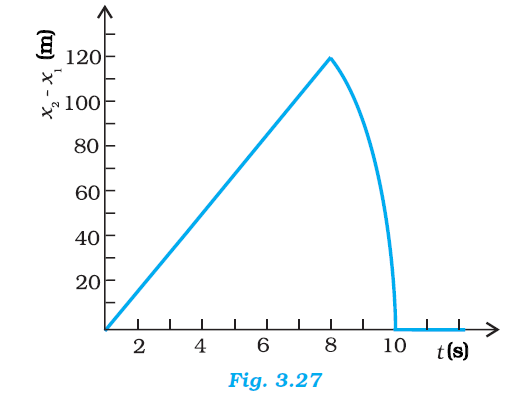## Pages

### Motion in a straight line NCERT Solutions Class 11 Physics - Solved Exercise Question 3.26

Question 3.26

Two stones are thrown up simultaneously from the edge of a cliff 200 m high with initial speeds of 15 m s-1 and 30 m s-1. Verify that the graph shown in Fig. 3.27 correctly represents the time variation of the relative position of the second stone with respect to the first. Neglect air resistance and assume that the stones do not rebound after hitting the ground. Take g = 10 m s-2. Give the equations for the linear and curved parts of the plot.Solution:

For first stone:
Initial velocity, uI = 15 m/s
Acceleration, a = –g = – 10 m/s2

Using the relation,
x1 = x0 + u1t + (1/2)at2
Where, height of the cliff, x0 = 200 m
x1 = 200 + 15t - 5t2      ......(i)
When this stone hits the ground, x1 = 0
∴– 5t2 + 15t + 200 = 0
t2 – 3t – 40 = 0
t2 – 8t + 5t – 40 = 0
t (t – 8) + 5 (t – 8) = 0
t = 8 s or t = – 5 s
Since the stone was projected at time t = 0, the negative sign before time is meaningless.
t = 8 s
For second stone:
Initial velocity, uII = 30 m/s
Acceleration, a = – g = – 10 m/s2

Using the relation,
x2 = x0 + uIIt + (1/2)at2
= 200 + 30t - 5t2........(ii)
At the moment when this stone hits the ground; x2 = 0
– 5t2 + 30 t + 200 = 0
t2 – 6t – 40 = 0
t2 – 10t + 4t + 40 = 0
t (t – 10) + 4 (t – 10) = 0
t (t – 10) (t + 4) = 0
t = 10 s or t = – 4 s
Here again, the negative sign is meaningless.
t = 10 s
Subtracting equations (i) and (ii), we get
x2 - x1 = (200 + 30t -5t2) - (200 + 15t -5t2)
x2 - x1 =15t     ....... (iii)
Equation (iii) represents the linear path of both stones. Due to this linear relation between (x2 x1) and t, the path remains a straight line till 8 s.
Maximum separation between the two stones is at t = 8 s.
(x2x1)max = 15× 8 = 120 m
This is in accordance with the given graph.
After 8 s, only second stone is in motion whose variation with time is given by the quadratic equation:
x2x1 = 200 + 30t – 5t2
Hence, the equation of linear and curved path is given by
x2 x1 = 15t (Linear path)
x2 ­– x1 = 200 + 30t – 5t2 (Curved path)